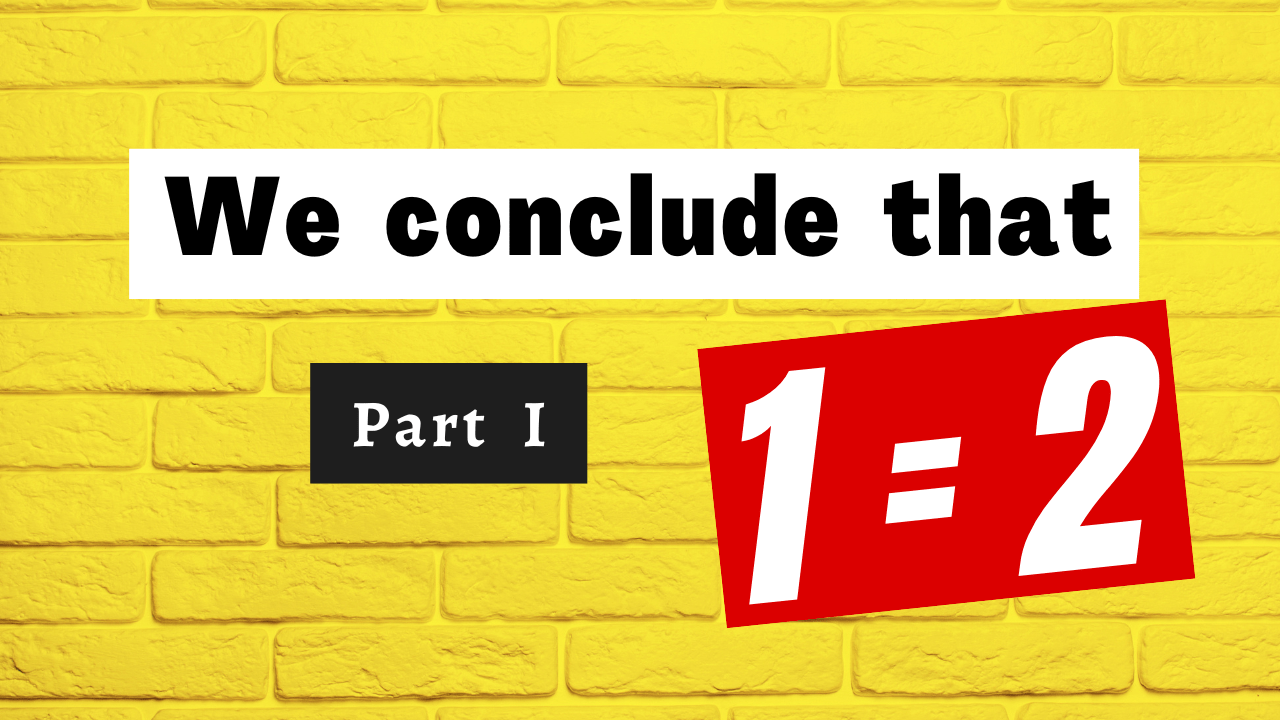## We conclude that 1 = 2 – First part

I often say that when you can measure what you are speaking about, and express it in numbers, you know something about it; but when you cannot measure it, when you cannot express it in numbers, your knowledge is of a meagre and unsatisfactory kind.

Lord Kelvin

Glad you came by. I wanted to let you know I appreciate your spending time here at the blog very much. I do appreciate your taking time out of your busy schedule to check out Math1089!

Suppose, you took your meal but your friends are expecting to share one more meal with them! After buying 1 kg of apple, the shopkeeper is asking you to pay the bills for 2 kg! Yesterday you borrowed a pen from your colleague and today, he is demanding back two! Imagine that your students came for the mathematics examination and once it got over, you instruct them to sit for the physics exam too.

Recall that, 1 is the starting number of the set of positive integers and 2 is the successor of it, hence the equality 1 = 2 is not possible. Despite of these, sometimes, somewhere, someone showed us that 1 = 2! It’s unfortunate, to have such instances. They are mathematical fallacy, an assumption (or series of steps) which is seemingly correct, though actually specious reasoning. Below, we will present a few such results, where due to some bugs, we can easily arrive at the conclusion 1 = 2.

It is against our known result, due to some error. But what is (are) that? Let’s ask “Is the division by 0 allowed?”. Of course, the answer is “NO”. But we did the same here! In step (e), while cancelling the factor yx, we ignored the fact that yx = xx = 0 in view of (a)! Though division by 0 is undefined and we did the same here, hence the surprising result.

We all know that 1 ≠ 2 but due to some invalid steps, we arrive at such conclusions. In fact, we should keep in mind that, x2 = y2 means x = ± y and not that x = y. Using this result religiously, we find that the error occurred while travelling from step (e) to (f).

Our first example was based on the mathematical philosophy that division by 0 is undefined. The second one remind us about the rules of taking square roots (from square quantities). The third example is from the world of complex numbers.

You might be tempted to ask that, how we can take the square root of a negative number? Using the square root of a negative number leads to the study of Complex Numbers. If so, then where is the flaw?

In step (c), we made the mistake. As a result, we got something unexpected. The next example from the domain of differentiation.

While taking the derivative of a function w.r.t. x, we need to take into account all  parts of that function which involve the variable. Note that, the number of times we addx to itself depends on x.

Our mistake was to only take the derivative with respect to the first variable, and ignore the second variable. Unsurprisingly, we made miracles happen after that. to continue with, let us consider the following example from integration community.

We can see these miracles only because of faulty steps. There are many such results from different parts of mathematics. Below we will consider two such interesting examples from infinite series.

If we take smaller number of terms and calculate the sum for few times, we find that the sum is actually approaching towards a specific number, which is ln 2 = 0.6931…. the same can also be viewed from the following diagram, where Sn is the sum of first n terms of the series..

To get rid from this problem, we have to look into the Riemann Rearrangement Theorem, which says that if an infinite series of real numbers is conditionally convergent, then its terms can be arranged in a permutation so that the new series converges to an arbitrary real number or diverges.

We can have many more results, establishing 1 = 2. In this document, the idea is to provide one example from each domain (the concept), so that whenever we use these results, we should take special care. Following are few more examples from different concepts of mathematics.

What is the flaw here? Is it some how related to the general solutions? Our next example involves symbol.

It looks like “The level of water in the Arabian sea remains same when someone is pouring one bottle of water and someone two!” Having the same water level, take away the Arabian sea from both sides!

Last but not the least, suppose on the contrary, that 1 = 2. Subtracting one from both sides and adding one to both sides, we get 0 = 1 and 2 = 3 respectively. If we again add one to both sides, give 3 = 4. Continuing in this way, we eventually find the chain 0 = 1 = 2 = 3 = 4 = …, which is of course not possible! The axiomatic approach of creating the set of positive integers states that a positive integer can never be equal to it’s successor!

That’s all in this blog. As the name suggests, there will be a second part of this article, will come under the heading We conclude that 1 = 2 – Second part.

Your suggestions are eagerly and respectfully welcome! See you soon with a new mathematics blog that you and I call Math1089 – Mathematics for All!“.